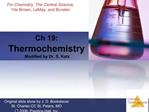DownloadDownload PresentationCh 19: Thermochemistry Modified by Dr. S. Katz

# Ch 19: Thermochemistry Modified by Dr. S. Katz

Download Presentation## Ch 19: Thermochemistry Modified by Dr. S. Katz

- - - - - - - - - - - - - - - - - - - - - - - - - - - E N D - - - - - - - - - - - - - - - - - - - - - - - - - - -
##### Presentation Transcript

1. Ch 19: Thermochemistry Modified by Dr. S. Katz

2. 1st Law of Thermodynamics Recall from Chapter 5 � Thermo (?H and ?E): �Energy cannot be created nor destroyed� It follows that EUniverse = constant. However� Energy can be� converted from one form to another. transferred between system and surroundings.

3. Spontaneous Processes Spontaneous processes are those that can proceed without any outside intervention. The gas in vessel B will spontaneously effuse into vessel A, but once the gas is in both vessels, it will not spontaneously

4. Spontaneous Processes Processes that are spontaneous in one direction are non-spontaneous in the reverse direction.

5. Spontaneous Processes Processes that are spontaneous at one temperature may be non-spontaneous at other temperatures. Above 0?C it is spontaneous for ice to melt. Below 0?C the reverse process is spontaneous.

11. Reversible Processes In a reversible process the system changes in such a way that the system and surroundings can be put back in their original states by exactly reversing the process.

12. Irreversible Processes Irreversible processes cannot be undone by exactly reversing the change to the system. Spontaneous processes are irreversible.

13. Entropy Entropy, S, can be thought of as a measure of the randomness of a system. It is related to the various modes of motion in molecules. Entropy is a ratio of heat delivered and the temperature at which it is delivered,

14. Entropy For a process occurring at constant temperature (an isothermal process), the change in entropy is equal to the heat that would be transferred if the process were reversible divided by the temperature:

19. Entropy Like total energy, E, and enthalpy, H, entropy is a state function. Therefore, ?S = Sfinal ? Sinitial so ?S� = ?n?S�(products) - ?m?S�(reactants)

20. 2nd Law of Thermodynamics The 2nd law states that the ?entropy of the universe increases for spontaneous processes and does not change for reversible processes. For reversible processes: ?Suniv = ?Ssystem + ?Ssurroundings = 0 For irreversible processes: ?Suniv = ?Ssystem + ?Ssurroundings > 0

21. Entropy on the Molecular Scale Entropy is based upon molecular motion - microstates. Molecules exhibit several types of motion: Translational: Movement of the entire molecule from one place to another. Vibrational: Periodic motion of atoms within a molecule. Rotational: Rotation of the molecule on about an axis or rotation about ? bonds. ??

22. Entropy on the Molecular Scale Entropy is essentially a mathematical combination of microstates, W, combined with a constant, S = k lnW where k is the Boltzmann constant, 1.38 ? 10?23 J/K, which you will never have to calculate. You may have to estimate relative entropies though.

23. Entropy on the Molecular Scale The number of microstates and, therefore, entropy, tends to increase with increases in Temperature. Volume. The number of independently moving molecules and atoms.

24. Entropy and Physical States Entropy increases with the freedom of motion of molecules, sometimes called degrees of freedom. Therefore, S(g) > S(l) > S(s)

25. Solutions Generally, when a solid is dissolved in a solvent, entropy increases.

26. Entropy Changes In general, entropy increases when Gases are formed from liquids and solids. Liquids or solutions are formed from solids. The number of gas molecules increases. The number of moles increases.

27. 3rd Law of Thermodynamics The entropy of a pure crystalline substance at absolute zero is 0.

28. Standard Entropies These are molar entropy values of substances in their standard states. Standard entropies tend to increase with increasing molar mass.

29. Standard Entropies Larger and more complex molecules have greater entropies.

32. Entropy Changes Entropy changes for a reaction can be estimated in a manner analogous to that by which ?H is estimated: ?S� = ?n?S�(products) - ?m?S�(reactants) where n and m are the coefficients in the balanced chemical equation.

35. System, Surrounding, and the Universe For an isothermal process (const T):

36. Gibbs Free Energy, ?G ?T?Suniv = ?Hsys ? T?Ssys ?TDSuniv is known as Gibbs Free Energy, ?G When ?Suniv is (+), ?G is (-). When ?G is (-), the process is spontaneous. ?G = ?H ? T?S ? ?G is a measure of the spontaneity of chemical processes ?

37. Gibbs Free Energy If DG is negative, the forward reaction is spontaneous. If DG is 0, the system is at equilibrium. If ?G is positive, the reaction is spontaneous in the reverse direction.

38. Standard ?G Changes ?G? is also a state function; standard free energies of formation values, ?Gf? can be found in the table in Appendix C.

40. Free Energy and Temperature At temperatures other than 25�C, DG� = DH? ? T?S? How does ?G? change with temperature? There are two parts to the free energy equation: ?H?� the enthalpy term T?S? � the entropy term The temperature dependence of free energy, then, comes from the entropy term.

41. Free Energy and Temperature

46. Free Energy and Equilibrium Under any conditions, standard or nonstandard, the free energy change can be found this way: ?G = ?G? + RT lnQ (Under standard conditions, all concentrations are 1 M, so Q = 1 and lnQ = 0; the last term drops out.)

47. Free Energy and Equilibrium At equilibrium, Q = K, and ?G = 0. The equation becomes 0 = ?G? + RT lnK Rearranging, this becomes ?G? = ?RT lnK or, K = e??G?/RT